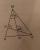Hexagon

There is regular hexagon ABCDEF. If area of the triangle ABC is 22, what is area of the hexagon ABCDEF?

I do not know how to solve it simply....

Result

S =  132

Solution:Leave us a comment of example and its solution (i.e. if it is still somewhat unclear...):Be the first to comment!Next similar examples:

1. Hexagon ACalculate area of regular hexagon inscribed in circle with radius r=9 cm.
2. Candy - MOGretel deploys to the vertex of a regular octagon different numbers from one to eight candy. Peter can then choose which three piles of candy give Gretel others retain. The only requirement is that the three piles lie at the vertices of an isosceles triang
3. TriangleCalculate the area of ​​the triangle ABC if b = c = 17 cm, R = 19 cm (R is the circumradius).
4. Right triangleRight triangle ABC with side a = 19 and the area S = 95. Calculate the length of the remaining sides.
5. SailsWe known heights 220, 165 and 132 of sail. It has triangular shape. What is the surface of the sail?
6. Rectangular trianglesThe lengths of corresponding sides of two rectangular triangles are in the ratio 2:5. At what ratio are medians relevant to hypotenuse these right triangles? At what ratio are the contents of these triangles? Smaller rectangular triangle has legs 6 and 8 c
7. The farmerThe farmer would like to first seed his small field. The required amount depends on the seed area. Field has a triangular shape. The farmer had fenced field, so he knows the lengths of the sides: 119, 111 and 90 meters. Find a suitable way to determine th
8. SAS triangleThe triangle has two sides long 7 and 19 and included angle 36°. Calculate area of this triangle.
9. Centre of massThe vertices of triangle ABC are from the line p distances 3 cm, 4 cm and 8 cm. Calculate distance from the center of gravity of the triangle to line p.
10. Euclid 5Calculate the length of remain sides of a right triangle ABC if a = 7 cm and height vc = 5 cm.
11. Obtuse angleThe line OH is the height of the triangle DOM, line MN is the bisector of angle DMO. obtuse angle between the lines MN and OH is four times larger than the angle DMN. What size is the angle DMO? (see attached image)
12. Right trianglesHow many right triangles we can construct from line segments 3,4,5,6,8,10,12,13,15,17 cm long? (Do not forget to the triangle inequality).
13. MapleMaple peak is visible from a distance 3 m from the trunk from a height of 1.8 m at angle 62°. Determine the height of the maple.
14. Theorem proveWe want to prove the sentence: If the natural number n is divisible by six, then n is divisible by three. From what assumption we started?
15. CandiesIn the box are 12 candies that look the same. Three of them are filled with nougat, five by nuts, four by cream. At least how many candies must Ivan choose to satisfy itself that the selection of two with the same filling? ?
16. Reference angleFind the reference angle of each angle:
17. AP - simpleDetermine the first nine elements of sequence if a10 = -1 and d = 4